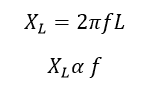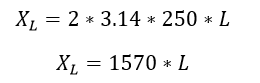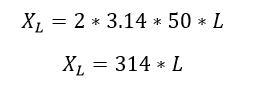# How Does Series Reactor Reduce Harmonic

### How Does Series Reactor Reduce Harmonic:

Harmonic is defined as integer multiplication of fundamental component. Harmonic is a very dangerous quantity in power system. It causes reduction of torque in induction motor, rising neutral voltage, conductor overloading etc. They are generated by the modern electronics loads such as battery charger, VFDs, all communication devices, etc . The frequency of the harmonic current or voltage is higher than the fundamental components. i.e second harmonic has 100 Hz, third harmonic has 150 Hz, fourth has 200 Hz like that. The higher order harmonic has higher frequency. Here most widely used harmonic filter is an inductor. As the property of inductor which is used as harmonic filter.

The inductive reactance is directly proportional to the frequency. Hence the inductor does not allow high frequency signals. So that the harmonic current gets reduced. Let see the theoretical explanation.For example, take 5th order harmonic and fundamental harmonic. Inductive reactance